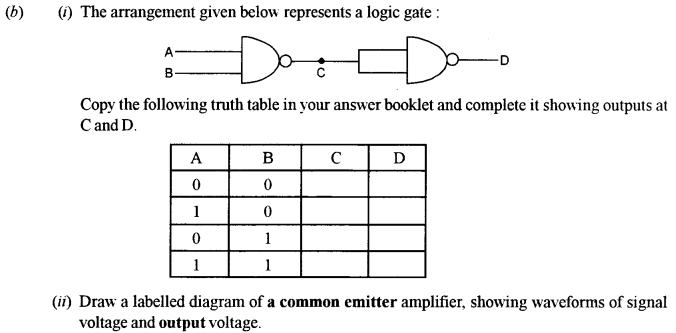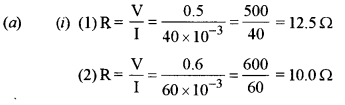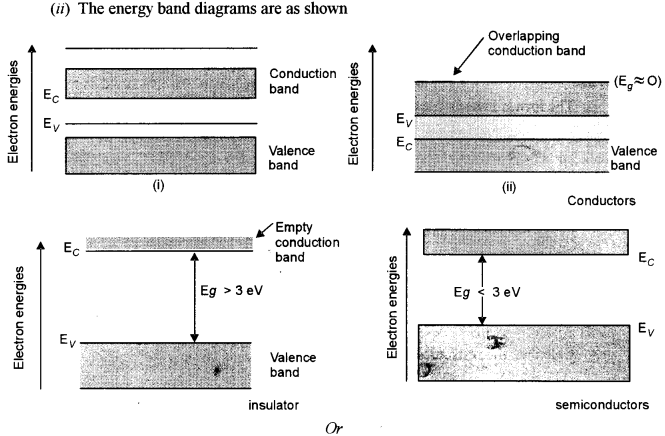## ISC Physics Previous Year Question Paper 2018 Solved for Class 12

Maximum Marks: 70
Time allowed: Three hours

• Candidates are allowed additional 15 minutes for only reading the paper. They must NOT start writing during this time.
• All questions are Compulsory.
• This question paper is divided into 4 sections, A, B, C and D as follows
• Section A: Question number 1 is of twelve marks. All parts of this question are compulsory.
• Section B: Question numbers 2 to 12 carry 2 marks each with two questions having internal choice.
• Section C: Question numbers 13 to 19 carry 3 marks each with two questions having internal choice.
• Section D: Question numbers 20 to 22 are long-answer type questions and carry 5 marks each.
• Each question has an internal choice.
• The intended marks for questions are given in brackets [ ].
• All working, including rough work, should be done on the same sheet as and
• Answers to sub parts of the same question must be given in one place only.
• A list of useful physical constants is given at the end of this paper.
• A simple scientific calculator without a programmable memory may be used for calculations.

Section – A

Question 1.
(A) Choose the correct alternative (a), (b), (c) or (d) for each of the questions given below : [5×1]
(i)The order of coloured rings in a carbon resistor is red, yellow, blue and silver. The resistance of the carbon resistor is
(a) 24 × 106 Ω ± 5%
(b) 24 × 106 Ω ± 10%
(c) 34 × 10Ω + 10%
(d) 26 × 104 Ω + 5%

(ii) A circular coil carrying a current I has radius R and number of turns N. If all the three, i.e., the current I, radius R and number of turns N are doubled, then, magnetic field at its center becomes:
(a) Double
(b) Half
(c) Four times
(d) One fourth

(iii) An object is kept on the principal axis of a concave mirror of focal length 10 cm, at a distance of 15 cm from its pole. The image formed by the mirror is:
(a) Virtual and magnified
(b) Virtual and diminished
(c) Real and magnified
(d) Real and diminished

(iv) Einstein’s photoelectric equation is :
(a) Emax = hλ – Φ0
(b) Emax = hc/λ – Φ0
(c) Emax = hv – Φ0
(d) Emax = hc/λ – Φ0
(v) In Bohr’s model of hydrogen atom, radius of the first orbit of an electron is r0. Then, radius of the third orbit is :
(a) r0/9
(b) r0
(c) 3r0
(d) 9r0

(B) Answer the following questions briefly and to the point [7 × 1]
(i) In a potentiometer experiment, balancing length is found to be 120 cm for a cell E1 of emf 2 V What will be the balancing length for another cell E2 of emf 1.5V? (No other changes are made in the experiment.)
(ii) How will you convert a moving coil galvanometer into a voltmeter ?
(iii) A moving charged particle q.travelling along the positive x-axis enters a uniform magnetic field B. When will the force acting on q be maximum ?
(iv) Why is the core of a transformer laminated ?
(v) Ordinary (i.e.. unpolarised) light is incident on the surface of a transparent material at the polarising angle. If it is partly reflected and partly refracted, what is the angle between the reflected and the refracted rays ?
(vi) Define coherent sources of light.
(vii) Name a material which is used in making control rods in a nuclear reactor.

(A)
(i) (c)
(ii) (a)
(iii) (c)
(iv) (b)
(v) (c)
(B) (i) L1 = 120 cm, E1 = 2v, L2 = ?, E2 = 1.5 V
Using $$\frac{E_{2}}{E_{1}}=\frac{L_{2}}{L_{1}} \Rightarrow L_{2}=\frac{1.5}{2} \times 120=90 \mathrm{cm}$$
(ii) A moving coil galvanometer can be converted into a voltmeter by connecting a very high resistance in series with the galvanometer.
(iii) The force experienced by the charge is given by F = Bqv sin θ. where θ is the angle between the velocity vector and the magnetic field. For maximum force θ = 90 °
(iv) It is laminated to reduce the effects of eddy currents.
(v) 90°
(vi) Coherent sources of light are those sources which have zero or constant phase difference.

Section – B

Question 2.
Define current density. Write an expression which connects current density with drift speed.
It is defined as the current flowing per unit area. The relation J = v ne.

Question 3. 
(a) A long horizontal wire P carries a current of 50 A. It is rigidly fixed. Another wire Q is placed directly above and parallel to P as shown in Figure 1 below. The weight per unit length of the wire Q is 0.025 N m-m and it carries a current of 25 A. Find the distance r of the wire Q from the wire P so that the wire Q remains at rest.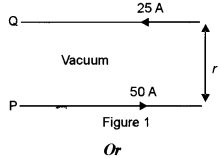(b) Calculate force per unit length acting on the wire B due to the current flowing in the wire A. (See figure 2 below):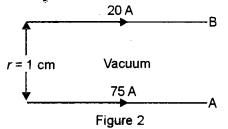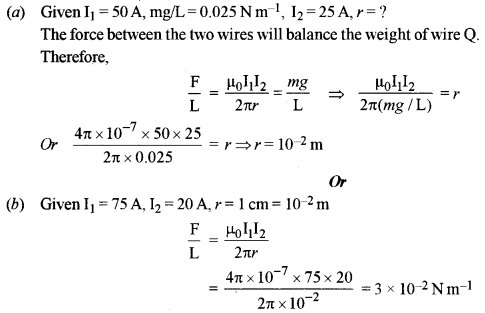Question 4. 
(i) Explain Curie’s law for a para magnetic substance.
(ii) A rectangular coil having 60 turns and area of 0.4 m2 is held at right angles to a uniform magnetic field of flux density’ 5 × 1o-5 T. Calculate the magnetic flux passing through it.
(i) According to Curie’s law, the magnetization in a para magnetic material is directly proportional to the applied magnetic field. If the object is heated, the magnetization is viewed to be inversely proportional to the temperature.
It can be framed into an equation
M = C × (B/T)
wherein, M = Magnetism,
B = Magnetic field (in tesla),
T = absolute temperature (in kelvin),
C = Curie constant
Curie’s law holds good for high temperature and not so strong magnetic fields.
(ii) $$\begin{array}{l}{\text { Given } n=60, \mathrm{A}=0.4 \mathrm{m}^{2}, \theta=0^{\circ}, \mathrm{B}=5 \times 10^{-5} \mathrm{T}, \phi=?} \\ {\phi=n \mathrm{BA} \cos \theta=60 \times 5 \times 10^{-5} \times 0.4 \times 1=1.2 \times 10^{-3} \mathrm{Wb}}\end{array}$$

Question 5.
What is motional emf ? State any two factors on which it depends. 
It is the emf induced in a coil when there is relative motion between the coil and the magnet. It depends upon
(i) Number of turns in the coil.
(ii) relative velocity between the coil and the magnet.

Question 6. 
(i) What is the ratio of the speed of gamma rays to that of radio waves in vacuum ?
(ii) Name an electromagnetic wave which is used in the radar system used in aircraft navigation.
(i) 1:1
(ii) Microwave

Question 7. 
A biconvex lens made of glass (refractive index 1.5) has two spherical surfaces having radii 20 cm and 30 cm. Calculate its focal length.
Given R1 = 20 cm, R2 = – 30 cm, n= 1.5, f= ?
Using lens makers formula, we have
$$\frac{1}{f}=(n-1)\left(\frac{1}{\mathrm{R}_{1}}-\frac{1}{\mathrm{R}_{2}}\right)=(1.5-1)\left(\frac{1}{20}-\frac{1}{-30}\right)=\frac{1}{24}$$
So f=24cm

Question 8. 
State any two differences between primary rainbow and secondary rainbow.

• Primary is smaller than secondary.
• Primary is darker than secondary.

Question 9. 
(i) State de Broglie hypothesis.
(ii) With reference to photoelectric effect, define threshold wavelength.
(i) It states that matter has dual nature.
(ii) It is the wavelength below which photoelectric effect takes place.

Question 10. 
Calculate the minimum wavelength of the spectral line present in Balmer series of hydrogen.
For the shortest wavelength (series limit ) nf = 2 and ni = ∝ such that the wavelength is
$$\frac{1}{\lambda}=\mathrm{R}_{\mathrm{H}}\left(\frac{1}{2^{2}}-\frac{1}{\infty}\right)=\frac{\mathrm{R}_{\mathrm{H}}}{4} \text { or } \lambda=3648 \mathrm{A}^{\circ}=\frac{4}{\mathrm{R}}$$

Question 11. 
(a) What is meant by pair annihilation ? Write a balanced equation for the same.
Or
(b) What is meant by the terms half-life of a radioactive substance and binding energy of a nucleus ?
(a) In the pair annihilation, the electron and positron in the stationary state combine with each other and annihilate. Surely, the particles are disappeared and radiation energy will occur instead of two particles.
K + K+ + 2m0c2 = 2 hv where K and K+ represent the kinetic energy of the electron and positron before the collision. Also, 2 m0c2 means the rest mass energy of both particles.
Or
(b) Half life: It is the time in which half the nuclei disintegrate.
Binding energy : It is the energy required to disintegrate a nucleus.

Question 12. 
In a communication system, what is meant by modulation? State any two types of modulation.
Modulation is the superimposition of a low frequency signal over a high frequency signal.
(i) Amplitude modulation
(ii) Frequency modulation

Section – C

Question 13. 
Obtain an expression for intensity of electric field at a point in end on position, i. e. axial position of an electric dipole.
Consider an electric dipole consisting of – q and + q charges separated by a distance 2a as shown in. figure below. Let P be the point of observation on the axial line where the electric field has to be found. Let it be at a distance r from the center O of the dipole. Let us suppose that the dipole is placed in vacuum.
Let EA and EB be the electric fields at point P due to the charges at A and B respectively. ThereforeQuestion 14. 
Deduce an expression for equivalent capacitance C when three capacitors C1. C2 and C3 are connected in parallel.
Consider two capacitors connected in parallel between points ‘a’ and b having capacitance C1, C2 and C3 as shown in figure. In this case the upper plates of the three capacitors are connected together by conducting wires to form an equipotential surface, and the lower plates form another. Hence in parallel combination the potential difference for all individual capacitors is same and is equal to V.Question 15. 
(a) ∈1 and ∈2 are two batteries having emf of 34 V and 10 V respectively and internal resistance of 1 Ω and 2 Ω respectively. They are connected as shown in Figure 3 below. Using Kirchhoff’s’ Laws of electrical networks, calculate the currents I1 and I2.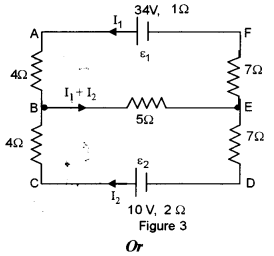(b) An electric bulb is marked 200 V, 100 W. Calculate electrical resistance of its filament. If five such bulbs are connected in series to a 200 V supply, how much current will flow through them ?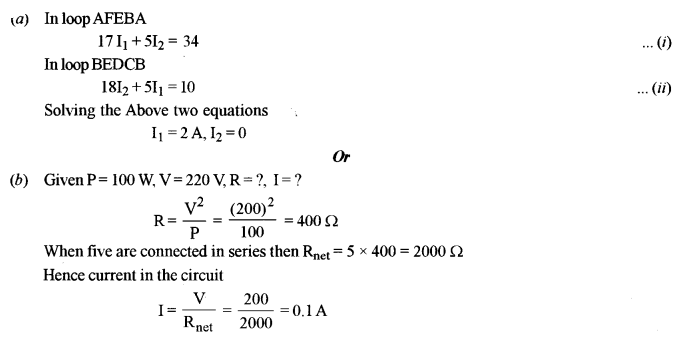Question 16.
(a) For any prism, prove that:
$$n=\frac{\sin \left(\frac{\mathrm{A}+\delta_{m}}{2}\right)}{\sin \left(\frac{\mathrm{A}}{2}\right)}$$
where the terms have their usual meaning.
Or
(b) When two thin lenses are kept in contact, prove that their combined or effective focal length F is given by:
$$\frac{1}{\mathrm{F}}=\frac{1}{f_{1}}+\frac{1}{f_{2}}$$
where the terms have their usual meaning.
(a) Consider a cross section XYZ of a prism as shown in figure as shown.(b) Consider two lenses A and B of focal length f1 and f2 placed in contact with each other. Let the object be placed at a point O beyond the focus of the first lens L1 as shown.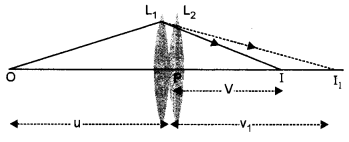The first lens L produces an image at I1. Since image I1 is real, it serves as a virtual object for the second lens L2, producing the final image at I. Since the lenses are thin, we assume the optical centers of the lenses to be coincident. Let this central point be denoted by P.
For the image formed by the first lens L1, we get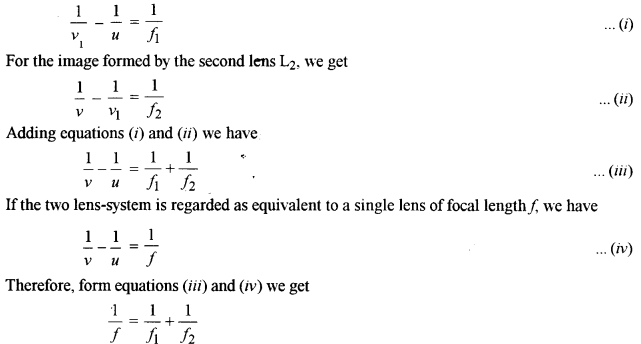Question 17. 
(i) In Young’s double slit experiment, show graphically how intensity of light varies with distance.
(ii) In Fraunhofer diffraction, how is the angular width of the central bright fringe affected when slit separation is increased ?
(i) The diagram is as shownQuestion 18.
Write one balanced equation each to show:
(i) Nuclear fission
(ii)Nuclear fusion
(iii) Emission of β (i. e. a negative beta particle)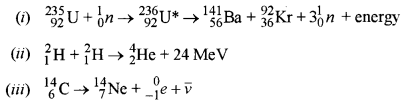Question 19. . 
With reference to semiconductor devices, define ap-type semiconductor and a Zener diode. What is the use of a Zener diode ?
A semiconductor which has more holes than electrons is called a p-type semiconductor. A Zener diode is a highly dope p-n junction which is operated in reverse bias. It is used as a voltage regulator.

Section – D

Question 20. 
(a) An alternating emf of 220 V is applied to a circuit containing a resistor R having resistance of 160 Ω and a capacitor C’ in series. The current is found to lead the supply voltage by an angle 0 = tan-1 (3/4).
(i) Calculate:
(1) The capacitive reactance
(2) Impedance of the circuit
(3) Current flowing in the circuit
(ii) If the frequency of the applied emf is 50 Hz. what is the value of the capacitance of the capacitor C’ ?
Or
(b) An ac generator generating an emf of ∈ = 300 sin (100 πt) V is connected to a series combination of 16 μF capacitor, 1H inductor and 100 Ω resistor.
Calculate:
(i) Impedance of the circuit at the given frequency.
(ii) Resonant frequency f0.
(iii) Power factor at resonant frequency f0.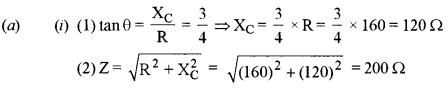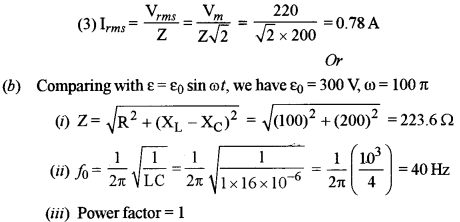Question 21. 
(a) Draw a labelled ray diagram of an image formed by a refracting telescope with Final image formed at infinity. Derive an expression for its magnifying power with the final image at infinity.
Or
(b) (i) Using Huygens’s wave theory, derive Snell’s law of refraction.
(ii) With the help of an experiment, state how will you identify whether a given beam of light is polarised or unpolarised.
(a) The labelled diagram is as shown below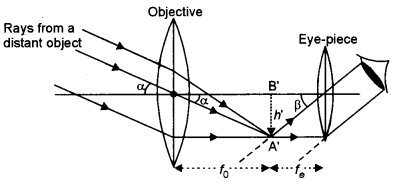The object subtends an angle a at the objective and would subtend essentially the same angle at the unaided eye. Also, since the observers’ eye is placed just to the right of the focal point F2‘, the angle subtended at the eye by the final image is very nearly equal to the angle p. Therefore,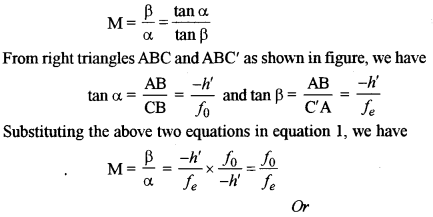(b) (i) Consider a plane surface KY separating a rarer medium of refractive index n1 from a denser medium of refractive index n2 Let c1 and c2 be the values of velocity of light in the two media. AB is a plane wave front incident on XY at an angle i. Let at a given instant the end A of wave front just strikes the surface XY but the other end B has still to cover a path BC. If it takes time t, then BC = c1t.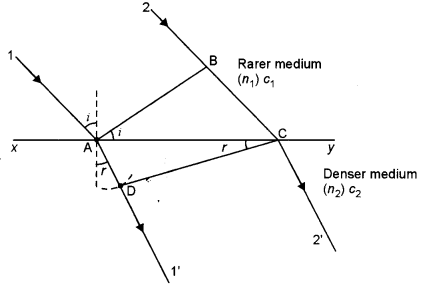According to Huygens’s principle point A meanwhile begins to act as secondary source of light and secondary wavelets from it will cover a distance c2t in second medium in time t. Draw a circular arc with A as center and c2t as radius and draw a tangent CD from point C on this arc. Then CD is the refracted wave front, which advances in the direction of rays 1,2. The refracted wave front subtends an angle r from surface XY.which is Snell’s law of refraction.
(ii) Figure shows an unpolarised light beam incident on the first polarizing sheet, called the polarizer where the pass axis is indicated by the straight line on the polarizer. The polarizer can be a thin sheet of tourmaline (a complex boro-silicate). The light, which is passing through this sheet, is polarized vertically as shown, where the transmitted electric vector is E0.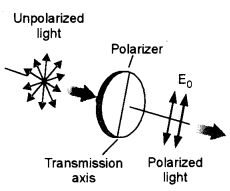When the polarizer is rotated two things can happen

(i) We will continue to get light. This shows that the incident light is unpolarized.
(ii) As we will rotate the polarizer the intensity of light coming out of it will diminish and for a certain angle no light will be obtained. This shows that the incident light if polarizer.

Question 22.
(a) (i) The forward characteristic curve of a junction diode is shown in Figure 4 below :